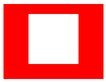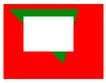Related Articles

# How to clear the canvas using clearRect in HTML ?

• Last Updated : 13 Mar, 2020

The clearRect() method of the Canvas 2D API which is used to erase the pixel in a rectangular area by setting the pixel color to transparent black (rgba(0, 0, 0, 0)).

Syntax:

`abc.clearRect(x, y, width, height);`

Paramaters:

• x, y: These paramter represents the top-left coordinate of the rectangular box.
• width: It is used to set the width of the rectangular box
• height: It is used to set the of the rectangular box

Example 1: In the following examplen the `clearRect()` method is used to clear the pixel of a rectangle of size (200×300) from (50, 20).

 ```<``html` `lang``=``"en"``>`` ` `<``head``>``    ``<``title``>``        ``How to clear the canvas``        ``using clearRect in HTML?``    `````` ` `<``body``>``    ``<``canvas` `id``=``"canvas"``>`` ` `    ``<``script``>``        ``const canvas = document.getElementById("canvas");``        ``const abc = canvas.getContext('2d');``     ` `        ``abc.beginPath();``        ``abc.fillStyle = "red";``        ``abc.fillRect(0, 0, 200, 300);``     ` `        ``abc.clearRect(50, 20, 100, 100);``    `````` ` ``

Output:Example 2:

 ```<``html` `lang``=``"en"``>`` ` `<``head``>``    ``<``title``>``        ``How to clear the canvas``        ``using clearRect in HTML?``    `````` ` `<``body``>``    ``<``canvas` `id``=``"canvas"``>`` ` `    ``<``script``>``        ``const canvas = document.getElementById("canvas");``        ``const abc = canvas.getContext('2d');``          ` `        ``// Draws a rectangle of 200x300``        ``abc.beginPath();``        ``abc.fillStyle = "red";``        ``abc.fillRect(0, 0, 200, 300);``          ` `        ``// Draws a traiangle``        ``abc.beginPath();``        ``abc.fillStyle = "green";``        ``abc.moveTo(10, 10);``        ``abc.lineTo(150, 10);``        ``abc.lineTo(120, 120);``        ``abc.closePath();``        ``abc.fill();``      ` `        ``abc.clearRect(40, 25, 100, 70);``    `````` ` ``

Output:Example 3: In this example we will erase whole canvas.

 ```<``html` `lang``=``"en"``>`` ` `<``head``>``    ``<``title``>``        ``How to clear the canvas``        ``using clearRect in HTML?``    `````` ` `<``body``>``    ``<``canvas` `id``=``"canvas"``>`` ` `    ``<``script``>``        ``const canvas = document.getElementById("canvas");``        ``const abc = canvas.getContext('2d');``      ` `        ``abc.beginPath();``        ``abc.fillStyle = "red";``        ``abc.fillRect(0, 0, 200, 300);``          ` `        ``// This line will erase whole canvas and``        ``// we will get an empty screen``        ``abc.clearRect(0, 0, canvas.width, canvas.height);``    `````` ` ``

Note: Be aware that `clearRect()` may cause unintended side effects if you’re not using paths properly. Make sure to call beginPath() before starting to draw new items after calling `clearRect()`.

Attention reader! Don’t stop learning now. Get hold of all the important HTML concepts with the Web Design for Beginners | HTML  course.

My Personal Notes arrow_drop_up